﻿ 基于囚徒困境策略的改进HK网络上的合作博弈
«上一篇文章快速检索 高级检索

 智能系统学报2018, Vol. 13Issue (3): 479-485  DOI: 10.11992/tis.2016120180

### 引用本文DENG Yunsheng, YANG Hongyong. Improved cooperative behavior in HK networks based on the prisoner dilemma game[J]. CAAI Transactions on Intelligent Systems, 2018, 13(3): 479-485. DOI: 10.11992/tis.201612018.### 文章历史

Improved cooperative behavior in HK networks based on the prisoner dilemma game
DENG Yunsheng, YANG HongyongSchool of Information and Electrical Engineering, Ludong University, Yantai 264025, China
Abstract: To simulate the cooperative behavior using the HK network model, we proposed a new adjustable power-law high-clustering network model to analyze the prisoner dilemma game. Through simulations, we investigated the effect of high clustering on the cooperative behavior. The experimental findings suggested that high clustering coefficient values may considerably contribute toward the emergence of cooperative behavior. We also found that with increasing temptation, the level of cooperation decreased and the variation was small. Altogether, the evolutionary game model promotes cooperation and hinders betrayal.
Key words: HK network    high clustering coefficient    adjustable power law    prisoner dilemma game    cooperative behavior    network game    temptation to betrayal    payoff matrix

1 网络博弈研究现状

2 改进的HK网络模型 2.1 网络模型内部演化机制

HK网络构造过程如下。

1)初始状态：网络初始状态有 ${m_0}$ 个全连通节点。

2)增长机制：每一个时间步，向网络中加入一个带有 $m$ 条边的节点 $i$ 。连接过程中，节点 $i$ 的第一条边按照度优先规则连接到网络中已存在的节点 $j$ ，即选择节点 $j$ 的概率为 ${\Pi _j} = {k_j}/\sum\limits_l {{k_l}}$

3)其余 $m - 1$ 条边以概率 $p$ 随机连接到节点 $j$ 的邻居上，否则以概率 $1 - p$ 使用度优先规则在网络中择优连接。

1)初始状态: 网络初始状态有 ${m_0}$ 个全连通节点。

2)增长机制: 每一个时间步，加入两个连接在一起的节点，每个节点有 $m$ 条边。这两个节点的每一条边进行两两配对，共有 $m$ 对边与网络中已经存在的节点进行相连。这两个节点之中的第一对边按度优先规则与网络中已存在节点(例如选中节点 $j$ )进行连接。

3)其余 $m - 1$ 对边，首先考虑以概率 $p$ 连接到节点 $j$ 的邻节点上。在此过程中，若有 ${\rm{len}} \geqslant m - 1$ ( ${\rm{len}}$ 为节点 $j$ 的邻居节点数目)，可将 $m - 1$ 对边随机无重复连接到 $i$ 的邻节点上，否则 $j$ 的邻节点全部与新加入的节点相连，多出的 $m - 1 - {\rm{len}}$ 对边在全局按照度优先规则进行连接。

4)若 $m - 1$ 对边未能以概率 $p$ 与节点 $j$ 的邻节点相连，则这 $m - 1$ 对边在全局按照度优先规则进行连接。

5)终止条件: 重复2)、3)、4)步，直至网络规模 $N$ 达到设定值。

2.2 EHK网络模型演化动力学分析

 $\begin{array}{*{20}{c}}{\displaystyle\frac{{{\rm{d}}{k_i}}}{{{\rm{d}}t}}} = {\displaystyle\frac{{2{k_i}}}{{\sum\limits_l {{k_l}} }} + \left( {2m - 2} \right)p\left( {\frac{{{k_{{j_1}}}\displaystyle\frac{1}{{{k_{{j_1}}}}}}}{{\sum\limits_l {{k_l}} }} + \frac{{{k_{{j_2}}}\displaystyle\frac{1}{{{k_{{j_2}}}}}}}{{\sum\limits_l {{k_l}} }} + \cdots + \displaystyle\frac{{{k_{{j_v}}}\displaystyle\frac{1}{{{k_{{j_v}}}}}}}{{\sum\limits_l {{k_l}} }}} \right)}+\\{ \left( {2m - 2} \right)\left( {1 - p} \right)\displaystyle\frac{{{k_i}}}{{\sum\limits_l {{k_l}} }} = 2m\frac{{{k_i}}}{{\sum\limits_l {{k_l}} }}}\end{array}$ (1)

 $\frac{{{\rm{d}}{k_i}}}{{{\rm{d}}t}} = 2m\frac{{{k_i}}}{{\sum\limits_l {{k_l}} }} = 2m\frac{{{k_i}}}{{\left( {4m + 2} \right)t}} = \frac{{m{k_i}}}{{\left( {2m + 1} \right)t}}$ (2)

 ${\rm{ln}}{k_i} = \frac{m}{{2m + 1}}{\rm{ln}}t + c$ (3)

 $c = {\rm{ln}}\frac{{m + 1}}{{t_i^{\frac{m}{{2m + 1}}}}},\;\;\;\;{k_i} = \left( {m + 1} \right){\left( {\frac{t}{{{t_i}}}} \right)^{\frac{m}{{2m + 1}}}}$ (4)

 $\begin{array}{*{20}{c}} {P\left( {{k_i} < K} \right)} = {P\left( {\left( {m + 1} \right){{\left( {\displaystyle\frac{t}{{{t_i}}}} \right)}^{\frac{m}{{2m + 1}}}} < K} \right)} = \\ {P\left( {{t_i} > \displaystyle\frac{t}{{{{\left( {\displaystyle\frac{k}{{m + 1}}} \right)}^{2m + 1}}}}} \right)} \end{array}$ (5)

 $\begin{array}{c}P\left( {{k_i} < K} \right) = P\left( {{t_i} > \displaystyle\frac{t}{{{{\left( {\displaystyle\frac{k}{{m + 1}}} \right)}^{2m + 1}}}}} \right) = \\1 - \displaystyle\frac{1}{{{m_0} + t}}\displaystyle\frac{t}{{{{\left( {\displaystyle\frac{k}{{m + 1}}} \right)}^{\frac{{2m + 1}}{m}}}}}\end{array}$ (6)

 ${p\left( k \right)} = {\displaystyle\frac{{{\rm{d}}P\left( {{k_i} < K} \right)}}{{{\rm{d}}k}}} = {\displaystyle\frac{{t\left( {2m + 1} \right){{\left( {m + 1} \right)}^{\LARGE\frac{{2m + 1}}{m}}}}}{{m\left( {{m_0} + t} \right)}}{k^{ - 3 - \frac{1}{m}}}}$ (7)

2.3 仿真分析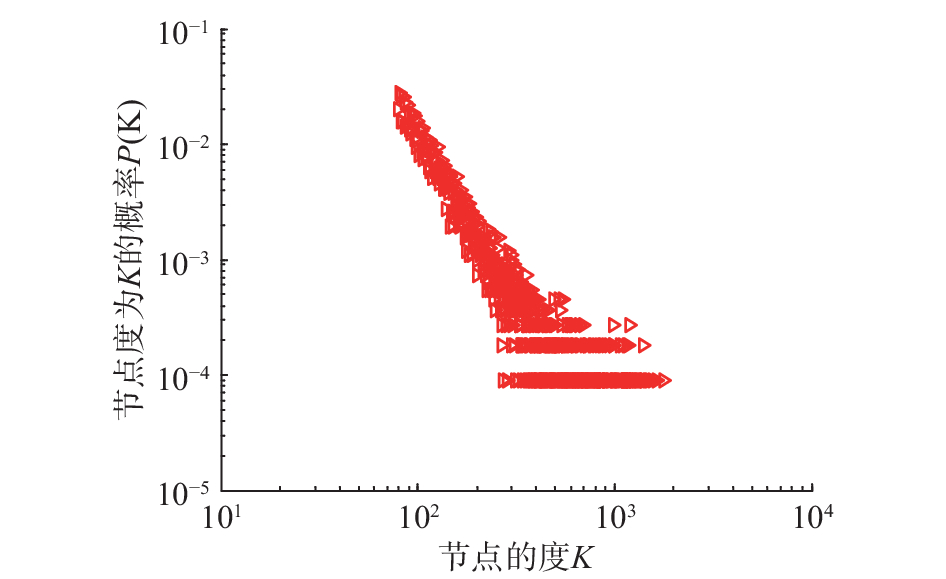Download: 图 1 EHK网络节点度分布图 Fig. 1 Degree distribution of EHK networks

 ${C_i} = \frac{{\text{包含节点}i\text{的三角形的数目}}}{{\text{以节点}i\text{为中心的连通三元组的数目}}}$ (8)

 ${\rm{CC}} = \frac{1}{N}\sum\limits_{i = 1}^N {{C_i}}$ (9)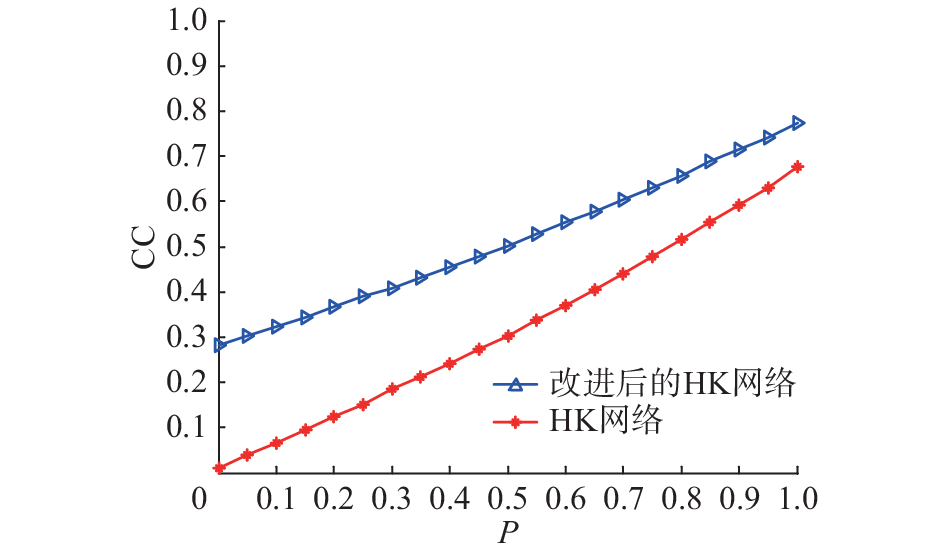Download: 图 2 EHK网络与HK网络平均聚类系数对比 Fig. 2 Comparison of the average clustering coefficient between EHK and HK

 $C(k) = \frac{{\sum\limits_i {{C_i}{\omega _{{k_i}k}}} }}{{\sum\limits_i {{\omega _{{k_i}k}}} }}$ (10)

 ${\omega _{{k_i}k}} = \left\{ {\begin{array}{*{20}{c}} {1,}&{{k_i} = k} \\ {0,}&{{k_i} \ne k} \end{array}} \right.$ (11)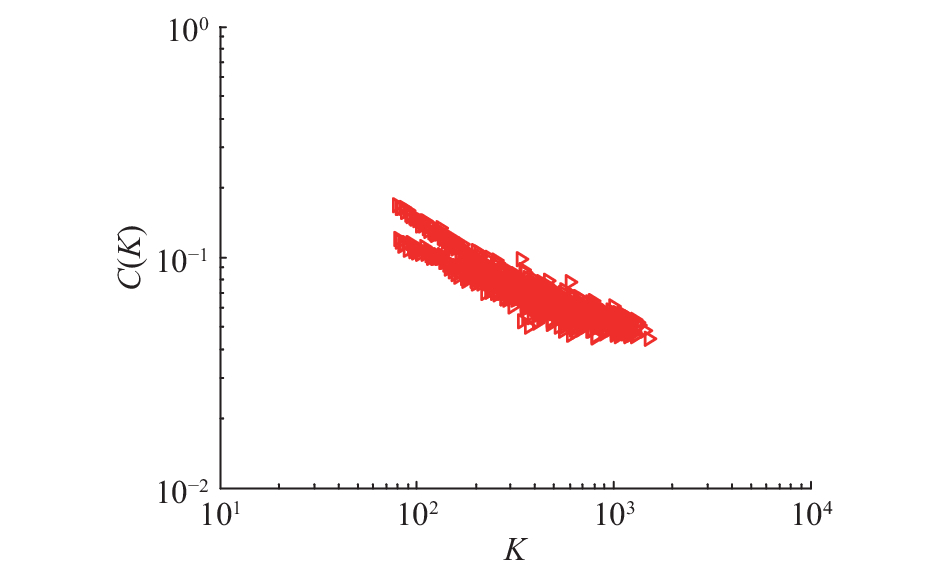Download: 图 3 平均聚类系数 $C\left( K \right)$ 与度的关系 Fig. 3 Relation between the average clustering coefficient and degree k

3 EHK网络上囚徒博弈 3.1 囚徒博弈模型

 $\begin{array}{*{20}{c}} {}&{\begin{array}{*{20}{c}} C&D \end{array}} \\ {\begin{array}{*{20}{c}} C \\ D \end{array}}&{\left[ {\begin{array}{*{20}{c}} {{R}}&{{S}} \\ {{T}}&{{P}} \end{array}} \right]} \end{array}$ (12)

 ${{P}} = \left[ {\begin{array}{*{20}{c}} 1&0 \\ b&0 \end{array}} \right]$ (13)

 ${U_i} = \sum\limits_{j \in {\varOmega _i}} {{{S}}_i^{\rm{T}}{{P}}{{{S}}_j}}$ (14)

 ${p_{i \leftarrow j}} = \frac{{{u_j} - {u_i}}}{{\left( {{{T}} - {{S}}} \right)\max \left( {{k_i},{k_j}} \right)}}$ (15)

3.2 仿真分析 3.2.1 高聚类特性对合作行为的影响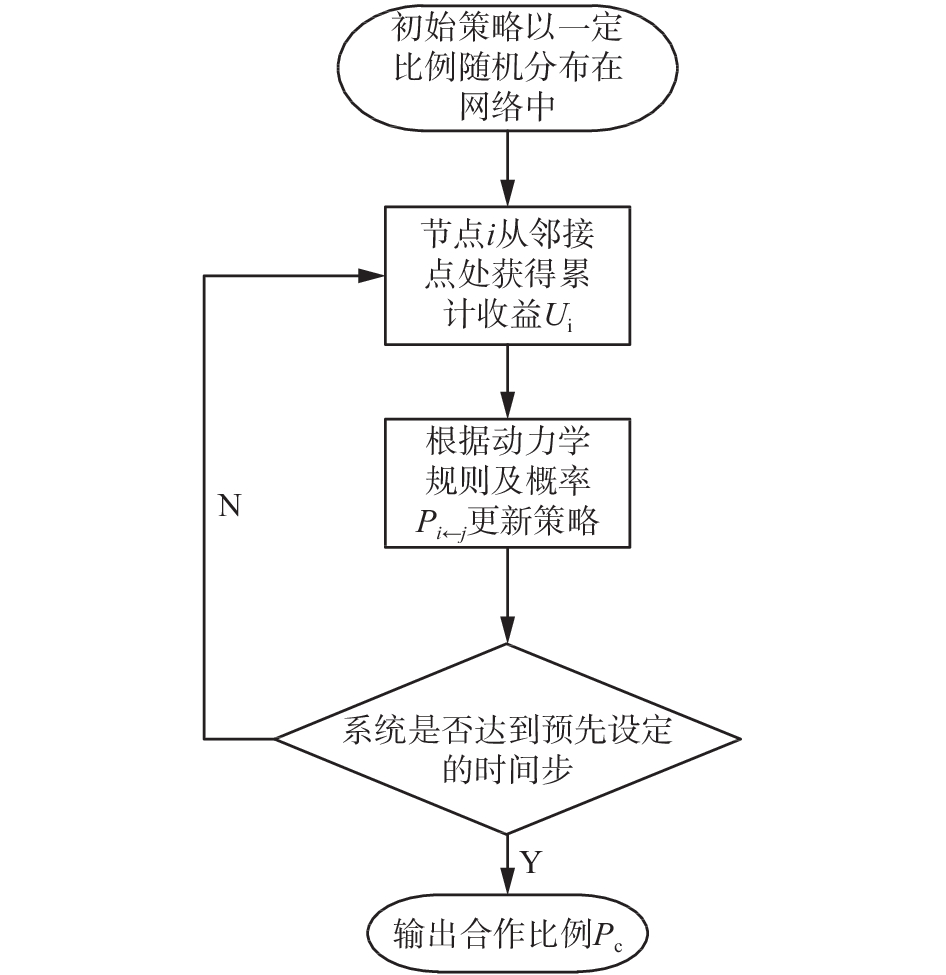Download: 图 4 博弈演化流程 Fig. 4 Process of game evolutionDownload: 图 5 合作者比例随聚类系数变化 Fig. 5 Variation in the partner proportion and clustering coefficient

3.2.2 背叛的诱惑对合作行为的影响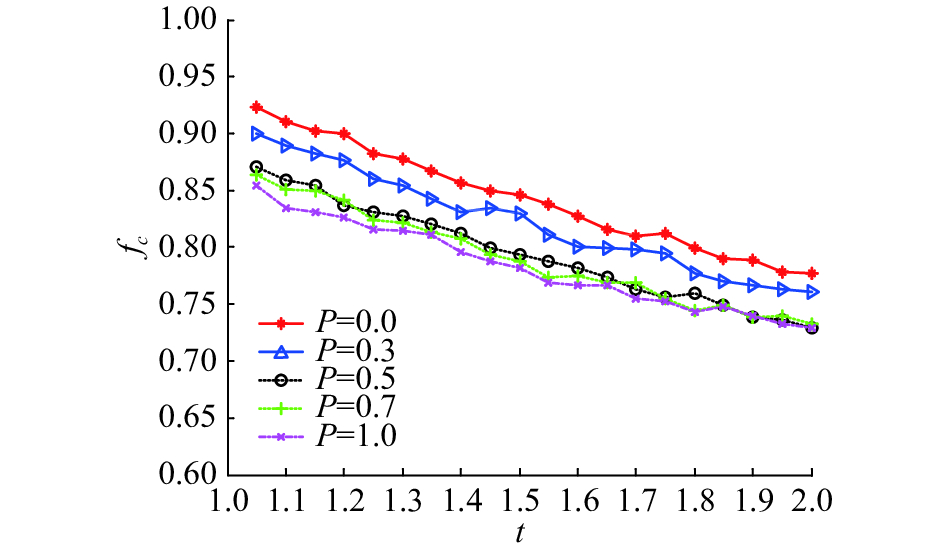Download: 图 6 合作者密度与欺骗诱惑关系 Fig. 6 Relationship between the partner density and deception temptation

4 结束语

  WATTS D J, STROGATZ S H. Collective dynamics of ‘small-world’ networks[J]. Nature, 1998, 393(6684): 440-442. DOI:10.1038/30918 (0)  BARABÁSI A L, ALBERT R, JEONG H. Mean-field theory for scale-free random networks[J]. Physica A: statistical mechanics and its applications, 1999, 272(1/2): 173-187. (0)  HOLME P, KIM B J. Growing scale-free networks with tunable clustering[J]. Physical review E, 2001, 65(2 Pt 2): 026107. (0)  李稳国, 王力虎, 陈明芳. HK网络演化模型的研究和改进[J]. 计算机工程, 2009, 35(3): 121-122, 125. LI Wenguo, WANG Lihu, CHEN Mingfang. Study and improvement on growing HK network model[J]. Computer engineering, 2009, 35(3): 121-122, 125. (0)  王丹, 井元伟, 郝彬彬. 扩展HK网络结构与同步能力的研究[J]. 物理学报, 2012, 61(22): 220511. WANG Dan, JING Yuanwei, HAO Binbin. Extended Holme-Kim network model and synchronizability[J]. Acta physica sinica, 2012, 61(22): 220511. DOI:10.7498/aps.61.220511 (0)  崔爱香, 傅彦. 加速增长的HK网络演化模型[J]. 计算机科学, 2015, 42(4): 37-39. CUI Aixiang, FU Yan. Accelerated-growth HK network evolution model[J]. Computer science, 2015, 42(4): 37-39. DOI:10.11896/j.issn.1002-137X.2015.04.005 (0)  徐玉珠, 张达敏, 曾成, 等. 改进HK网络演化模型的研究[J]. 电子科技, 2016, 29(3): 106-109. XU Yuzhu, ZHANG Damin, ZENG Cheng, et al. Research on and modeling of the improved HK network model[J]. Electronic science and technology, 2016, 29(3): 106-109. (0)  CHEN Liuqing, GAO Jinchun, XIE Gang, et al. Routing to enhance traffic capacity for scale-free networks with tunable clustering[C]//Proceedings of IEEE Advanced Information Technology, Electronic and Automation Control Conference. Chongqing, China, 2015: 110–113. (0)  VARGA I, KOCSIS G. Novel model of social networks with tunable clustering coefficient[C]//Proceedings of the 9th International Conference on Applied Informatics. Eger, Hungary, 2014: 171–176. (0)  ZHANG Xuejun, GU Bo, GUAN Xiangmin, et al. Cascading failure in scale-free networks with tunable clustering[J]. International journal of modern physics C, 2016, 27(8): 1650093. DOI:10.1142/S0129183116500935 (0)  LI Ruiqi, SUN Shiwen, MA Yilin, et al. Effect of clustering on attack vulnerability of interdependent scale-free networks[J]. Chaos, solitons & fractals, 2015, 80: 109-116. (0)  朱张祥, 刘咏梅. 在线社交网络谣言传播的仿真研究——基于聚类系数可变的无标度网络环境[J]. 复杂系统与复杂性科学, 2016, 13(2): 74-82. ZHU Zhangxiang, LIU Yongmei. Study of propagation of rumor in online social network based on scale-free network with tunable clustering[J]. Complex systems and complexity science, 2016, 13(2): 74-82. (0)  宋玉萍, 倪静. 网络集聚性对节点中心性指标准确性的影响[J]. 物理学报, 2016, 65(2): 028901. SONG Yuping, NI Jing. Effect of variable network clustering on the accuracy of node centrality[J]. Acta physica sinica, 2016, 65(2): 028901. (0)  NOWAK M A, MAY R M. Evolutionary games and spatial chaos[J]. Nature, 1992, 359(6398): 826-829. DOI:10.1038/359826a0 (0)  ASSENZA S, GÓMEZ-GARDEÑES J, LATORA V. Enhancement of cooperation in highly clustered scale-free networks[J]. Physical review E, 2008, 78(1 Pt 2): 017101. (0)  张文明. 网络演化博弈模型和仿真研究[D]. 哈尔滨: 哈尔滨工业大学, 2014. ZHANG Wenming. Model and simulation research on netwokded evolutionary games[D]. Harbin: Harbin Institute of Technology, 2014. (0)  LI Pingping, KE Jianhong, LIN Zhenquan, et al. Cooperative behavior in evolutionary snowdrift games with the unconditional imitation rule on regular lattices[J]. Physical review E, 2012, 85(2 Pt 1): 021111. (0)  ZHANG Wen, XU Chen, HUI P M. Spatial structure enhanced cooperation in dissatisfied adaptive snowdrift game[J]. European physical journal B, 2013, 86(5): 196. DOI:10.1140/epjb/e2013-30997-2 (0)  LUO Chao, ZHANG Xiaolin, LIU Hong, et al. Cooperation in memory-based prisoner’s dilemma game on interdependent networks[J]. Physica A: statistical mechanics and its applications, 2016, 450: 560-569. DOI:10.1016/j.physa.2016.01.032 (0)  MENG Xiaokun, SUN Shiwen, LI Xiaoxuan, et al. Interdependency enriches the spatial reciprocity in prisoner’s dilemma game on weighted networks[J]. Physica A: statistical mechanics and its applications, 2016, 442: 388-396. DOI:10.1016/j.physa.2015.08.031 (0)  向海涛, 梁世东. 双复杂网络间的演化博弈[J]. 物理学报, 2015, 64(1): 018902. XIANG Haitao, LIANG Shidong. Evolutionary gambling dynamics for two growing complex networks[J]. Acta physica sinica, 2015, 64(1): 018902. (0)